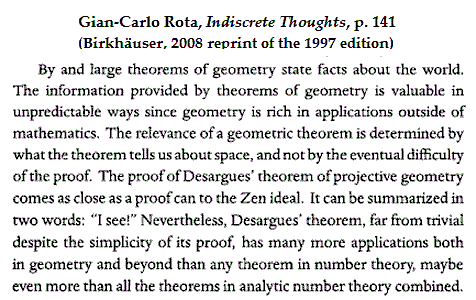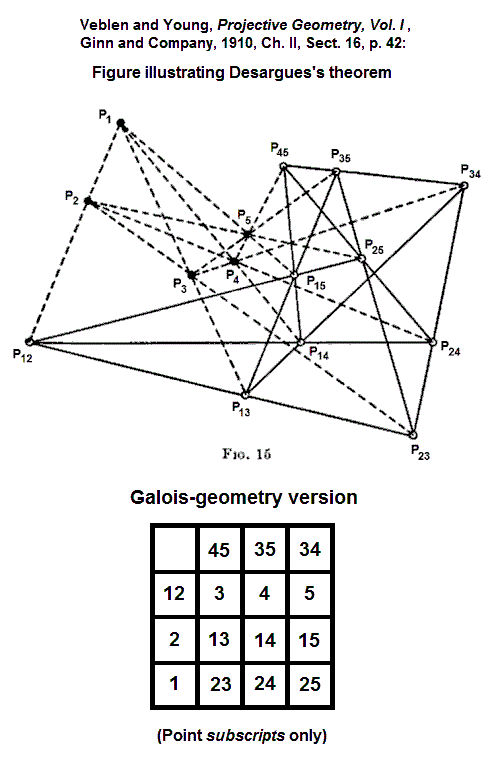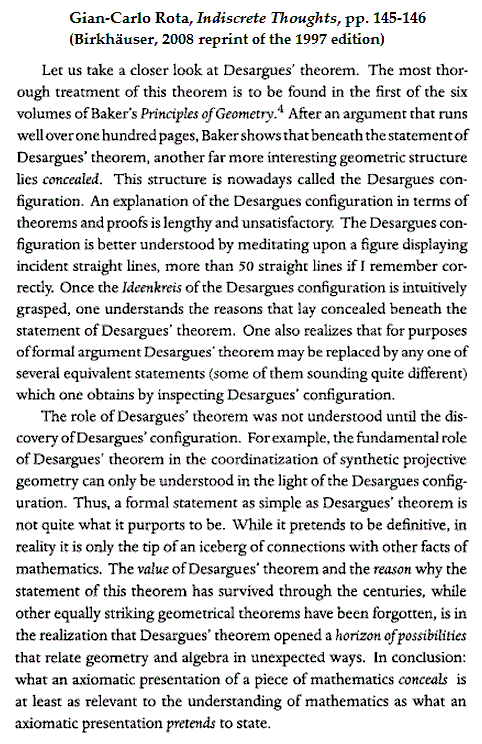# Log24

## Monday, April 1, 2013

### Desargues via Rosenhain

Filed under: General,Geometry — Tags: , — m759 @ 6:00 PM

Background: Rosenhain and Göpel Tetrads in PG(3,2)

 Introduction: The Large Desargues Configuration Added by Steven H. Cullinane on Friday, April 19, 2013 Desargues' theorem according to a standard textbook: "If two triangles are perspective from a point they are perspective from a line." The converse, from the same book: "If two triangles are perspective from a line they are perspective from a point." Desargues' theorem according to Wikipedia  combines the above statements: "Two triangles are in perspective axially  [i.e., from a line] if and only if they are in perspective centrally  [i.e., from a point]." A figure often used to illustrate the theorem,  the Desargues configuration , has 10 points and 10 lines, with 3 points on each line and 3 lines on each point. A discussion of the "if and only if" version of the theorem in light of Galois geometry requires a larger configuration— 15 points and 20 lines, with 3 points on each line  and 4 lines on each point. This large  Desargues configuration involves a third triangle, needed for the proof   (though not the statement ) of the  "if and only if" version of the theorem. Labeled simply "Desargues' Theorem," the large  configuration is the frontispiece to Volume I (Foundations)  of Baker's 6-volume Principles of Geometry . Point-line incidence in this larger configuration is, as noted in the post of April 1 that follows this introduction, described concisely  by 20 Rosenhain tetrads  (defined in 1905 by R. W. H. T. Hudson in Kummer's Quartic Surface ). The third triangle, within the larger configuration, is pictured below.A connection discovered today (April 1, 2013)—

Update of April 18, 2013

Note that  Baker's Desargues-theorem figure has three triangles,
ABC, A'B'C', A"B"C", instead of the two triangles that occur in
the statement of the theorem. The third triangle appears in the
course of proving, not just stating, the theorem (or, more precisely,
its converse). See, for instance, a note on a standard textbook for
further details.

(End of April 18, 2013 update.)

Update of April 14, 2013

See Baker's Proof (Edited for the Web) for a detailed explanation
of the above picture of Baker's Desargues-theorem frontispiece.

(End of April 14, 2013 update.)

Update of April 12, 2013

A different figure, from a site at National Tsing Hua University,
shows the three triangles of Baker's figure more clearly:

(End of update of April 12, 2013)

Update of April 13, 2013

Another in a series of figures illustrating
Desargues's theorem in light of Galois geometry:(End of update of April 13, 2013)Rota's remarks, while perhaps not completely accurate, provide some context
for the above Desargues-Rosenhain connection.  For some other context,
see the interplay in this journal between classical and finite geometry, i.e.
between Euclid and Galois.

For the recent  context of the above finite-geometry version of Baker's Vol. I
frontispiece, see Sunday evening's finite-geometry version of Baker's Vol. IV
frontispiece, featuring the Göpel, rather than the Rosenhain, tetrads.

For a 1986 illustration of Göpel and Rosenhain tetrads (though not under
those names), see Picturing the Smallest Projective 3-Space.

In summary… the following classical-geometry figures
are closely related to the Galois geometry PG(3,2):

 Volume I of Baker's Principles   has a cover closely related to  the Rosenhain tetrads in PG(3,2) Volume IV of Baker's Principles  has a cover closely related to the Göpel tetrads in PG(3,2) Foundations (click to enlarge) Higher Geometry (click to enlarge)